# Sources of Power Penalty

The sensitivity of the optical receiver in a realistic lightwave system is affected by several physical phenomena which, in combination with fiber dispersion, degrade the SNR at the decision circuit. Among the phenomena that degrade the receiver sensitivity are modal noise, dispersion broadening and intersymbol interference, mode-partition noise, frequency chirp, and reflection feedback. In this tutorial, we discuss how the system performance is affected by fiber dispersion by considering the extent of power penalty resulting from these phenomena.

#### 1. Modal Noise

Modal noise is associated with multimode fibers and was studied extensively during the 1980s. Its origin can be understood as follows. Interference among various propagating modes in a multimode fiber creates a speckle pattern at the photodetector.  The nonuniform intensity distribution associated with the speckle pattern is harmless in itself, as the receiver performance is governed by the total power integrated over the detector area. However, if the speckle pattern fluctuates with time, it will lead to fluctuations in the received power that would degrade the SNR. Such fluctuations are referred to as modal noise. They invariably occur in multimode fiber links because of mechanical disturbances such as vibrations and microbends. In addition, splices and connectors act as spatial filters. Any temporal changes in spatial filtering translate into speckle fluctuations and enhancement of the modal noise.  Modal noise is strongly affected by the source spectral bandwidth Δν since mode interference occurs only if the coherence time (Tc ≈ 1/Δν) is longer than the intermodal delay time ΔT given byFor LED-based transmitters Δν is large enough (Δν ~ 5 THz) that this condition is not satisfied. Most lightwave systems that use multimode fibers also use LEDs to avoid the modal-noise problem.

Modal noise becomes a serious problem when semiconductor lasers are used in combination with multimode fibers. Attempts have been made to estimate the extent of sensitivity degradation induced by modal noise by calculating the BER after adding modal noise to the other sources of receiver noise. The following figure shows the power penalty at a BER of 10-12 calculated for a 1.3-μm lightwave system operating at 140 Mb/s.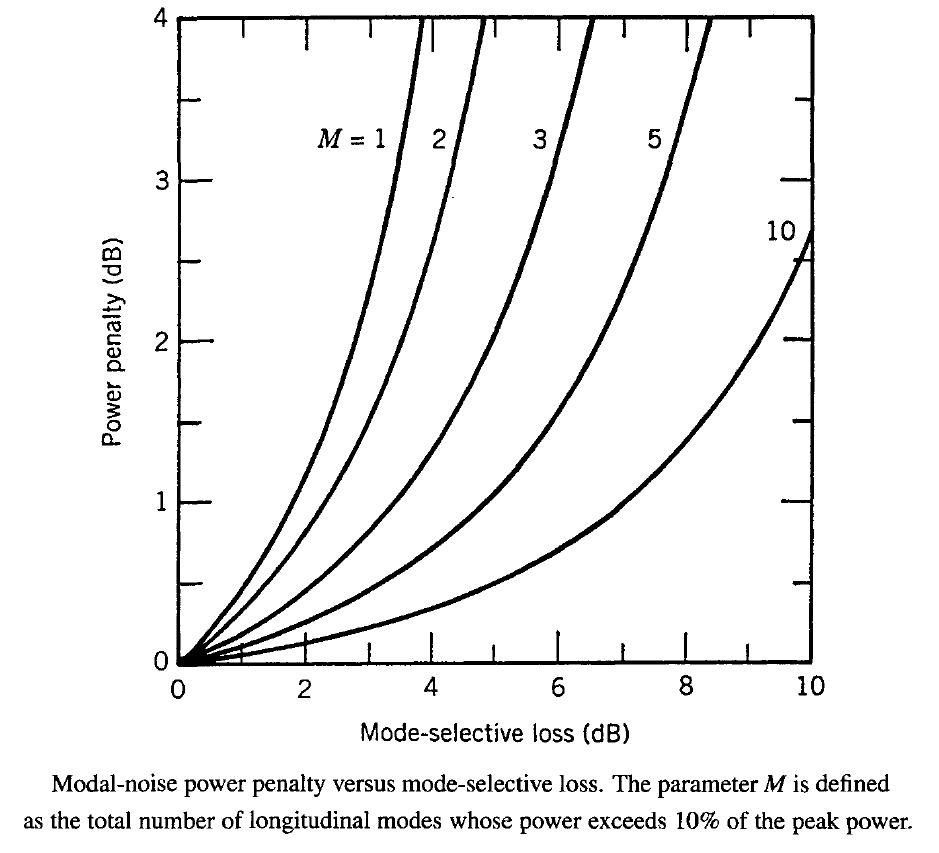The graded-index fiber has a 50-μm core diameter and supports 146 modes. The power penalty depends on the mode-selective coupling loss occurring at splices and connectors. It also depends on the longitudinal-mode spectrum of the semiconductor laser. As expected, power penalty decreases as the number of longitudinal modes increases because of a reduction in the coherence time of the emitted light.

Modal noise can also occur in single-mode systems if short sections of fiber are installed between two connectors or splices during repair or normal maintenance. A higher-order mode can be excited at the fiber discontinuity occurring at the first splice and then converted back to the fundamental mode at the second connector or splice. Since a higher-order mode cannot propagate far from its excitation point, this problem can be avoided by ensuring that the spacing between two connectors or splices exceeds 2 meter. Generally speaking, modal noise is not a problem for properly designed and maintained single-mode fiber-optic communication systems.

With the development of the vertical-cavity surface-emitting laser (VCSEL), the modal-noise issue has resurfaced in recent years. The use of such lasers in short-haul optical data links, making use of multimode fibers (even those made of plastic), is of considerable interest because of of the high bandwidth associated with VCSELs. Indeed, rates of several gigabits per second have been demonstrated in laboratory experiments with plastic fibers. However, VCSELs have a long coherence length as they oscillate in a single longitudinal mode. In a 1994 experiment the BER measurements showed an error floor at a level of 10-7 even when the mode-selective loss was only 1 dB. This problem can be avoided to some extent by using larger-diameter VCSELs which oscillate in several transverse modes and thus have a shorter coherence length. Computer models are generally used to estimate the power penalty for optical data links under realistic operating conditions. Analytic tools such the saddle-point method can also provide a reasonable estimate of the BER.

#### 2. Mode-Partition Noise

Multimode semiconductor lasers exhibit mode-partition noise (MPN), a phenomenon occurring because of an anticorrelation among pairs of longitudinal modes. In particular, various longitudinal modes fluctuate in such a way that individual modes exhibit large intensity fluctuations even though the total intensity remains relatively constant. MPN would be harmless in the absence of fiber dispersion, as all modes would remain synchronized during transmission and detection. In practice, different modes become unsynchronized, since they travel at slightly different speeds inside the fiber because of group-velocity dispersion. As a result of such desynchronization, the receiver current exhibits additional fluctuations, and the SNR at the decision circuit becomes worse than that expected in the absence of MPN. A power penalty must be paid to improve the SNR to the same value that is necessary to achieve the required BER. The effect of MPN on system performance has been studied extensively for multimode semiconductor lasers.

In the case of multimode semiconductor lasers, the power penalty can be calculated and is given by

δmpn = -5 log10(1 - Q2r2mpn)

where rmpn is the relative noise level of the received power in the presence of MPN. A simple model for estimating the parameter rmpn assumes that laser modes fluctuate in such a way that the total power remains constant under CW operation. It also assumes that the average mode power is distributed according to a Gaussian distribution of RMS width σλ and that the pulse shape at the decision circuit of the receiver is described by a cosine function. Different laser modes are assumed to have the same cross-correlation coefficient γcc, i.e.,for all i and j such that i ≠ j. The angular brackets denote an average over power fluctuations associated with mode partitioning. A straightforward calculation shows that rmpn is given by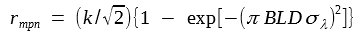where the mode-partition coefficient k is related to γcc as. The model assumes that mode partition can be quantified in terms of a single parameter k with values in the range 0-1. The numerical value of k is difficult to estimate and is likely to vary from laser to laser. Experimental measurements suggest that the values of k are in the range 0.6-0.8 and vary for different mode pairs.

The δmpn equation and the rmpn equation above can be used to calculate the MPN-induced power penalty. The following figure shows the power penalty at a BER of 10-9 (Q = 6) as a function of the normalized dispersion parameter BLDσλ for several values of the mode-partition coefficient k.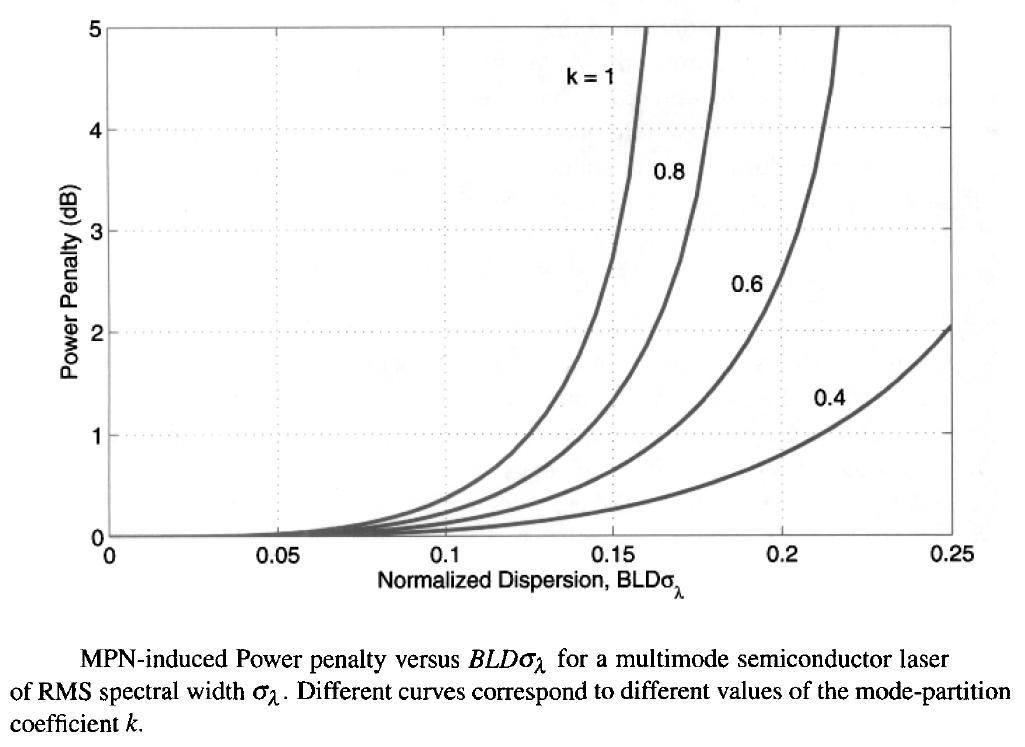For any value of k, δmpn increases rapidly with an increase in BLDσλ and becomes infinite when BLDσλ reaches a critical value. For k > 0.5, the MPN-induced power penalty becomes quite large if BLDσλ exceeds 0.15. However, it can be reduced to a negligible level (δmpn < 0.5 dB) by designing the optical communication system such that BLDσλ < 0.1. As an example, consider a 1.3-μm lightwave system. If we assume that the operating wavelength is matched to the zero-dispersion wavelength to within 10 nm, D ≈ 1 ps/(km-nm). A typical value of σλ for multimode semiconductor lasers is 2 nm. The MPN-induced power penalty would be negligible if the BL product were below 50 (Gb/s)-km. At B = 2 Gb/s the transmission distance is then limited to 25 km. The situation becomes worse for 1.55-μm lightwave systems for which D ≈ 16 ps/(km-nm) unless dispersion-shifted fibers are used. In general, the MPN-induced power penalty is quite sensitive to the spectral bandwidth of the multimode laser and can be reduced by reducing the bandwidth. In one study, a reduction in the carrier lifetime from 340 to 130 ps, realized by p-doping of the active layer, reduced the bandwidth of the 1.3-μm semiconductor lasers by only 40% (from 5.6 to 3.4 nm), but the power penalty decreased from an infinite value (BER floor above 10-9 level) to a mere 0.5 dB.

#### 3. Reflection Feedback and Noise

In most fiber-optic communication systems, some light is invariably reflected back because of refractive-index discontinuities occurring at splices, connectors, and fiber ends. The effects of such unintentional feedback have been studied extensively -  because they can degrade the performance of lightwave systems considerably. Even a relatively small amount of optical feedback affects the operation of semiconductor lasers and can lead to excess noise in the transmitter output. Even when an isolator is used between the transmitter and the fiber, multiple reflections between splices and connectors can generate additional intensity noise and degrade receiver performance. This subsection is devoted to the effect of reflection-induced noise on receiver sensitivity.

Most reflections in a fiber link originate at glass-air interfaces whose reflectivity can be estimated by using Rf = (nf - 1)2/(nf + 1)2, where nf is the refractive index of the fiber material. For silica fibers Rf = 3.6% (-14.4 dB) if we use nf = 1.47. This value increases to 5.3% for polished fiber ends since polishing can create a thin surface layer with a refractive index of about 1.6. In the case of multiple reflections occurring between two splices or connectors, the reflection feedback can increase considerably because the two reflecting surfaces act as mirrors of a Fabry-Perot interferometer. When the resonance condition is satisfied, the reflectivity increases to 14% for unpolished surfaces and to over 22% for polished surfaces. Clearly, a considerable fraction of the signal transmitted can be reflected back unless precautions are taken to reduce the optical feedback. A common technique is to use index-matching oil or gel near glass-air interfaces. Sometimes the tip of the fiber is curved or cut at an angle so that the reflected light deviates from the fiber axis. Reflection feedback can be reduced to below 0.1% by such techniques.

Semiconductor lasers are extremely sensitive to optical feedback; their operating characteristics can be affected by feedback as small as -80 dB. The most dramatic effect of feedback is on the fiber linewidth, which can narrow or broaden by several order of magnitude, depending on the exact location of the surface where feedback originates. The reason behind such a sensitivity is related to the fact that the phase of the reflected light can perturb the laser phase significantly even for relatively weak feedback levels. Such feedback-induced phase changes are detrimental mainly for coherent communication systems. The performance of direct-detection lightwave systems is affected by intensity noise rather than phase noise.

Optical feedback can increase the intensity noise significantly. Several experiments have shown a feedback-induced enhancement of the intensity noise occurring at frequencies corresponding to multiples of the external-cavity mode spacing. In fact, there are several mechanisms through which the relative intensity noise (RIN) of a semiconductor laser can be enhanced by the external optical feedback. In a simple model, the feedback-induced enhancement of the intensity noise is attributed to the onset of multiple, closely spaced, external-cavity longitudinal modes whose spacing is determined by the distance between the laser output facet and the glass-air interface where feedback originates. The number and the amplitudes of the external-cavity modes depend on the amount of feedback. In this model, the RIN enhancement is due to intensity fluctuations of the feedback-generated side modes. Another source of RIN enhancement has its origin in the feedback-induced chaos in semiconductor lasers. Numerical simulations of the rate equations show that the RIN can be enhanced by 20 dB or more when the feedback level exceeds a certain value. Even though the feedback-induced chaos is deterministic in nature, it manifests as an apparent RIN increase.

Experimental measurements of the RIN and the BER in the presence of optical feedback confirm that the feedback-induced RIN enhancement leads to a power penalty in lightwave systems. The figure below shows the result of the BER measurements for a VCSEL operating at 958 nm. Such a laser operates in a single longitudinal mode because of an ultrashort cavity length (~1 μm) and exhibits a RIN near -130 dB/Hz in the absence of reflection feedback. However, the RIN increases by as much as 20 dB when the feedback exceeds the -30-dB level. The BER measurements at a bit rate of 500 Mb/s shows a power penalty of 0.8 dB at a BER of 10-9 for -30-dB feedback, and the penalty increases rapidly at higher feedback levels.The power penalty can be calculated by following the analysis of another tutorial and is given by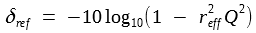where reff is the effective intensity noise over the receiver bandwidth Δf and is obtained from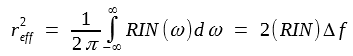In the case of feedback-induced external-cavity modes, reff can be calculated by using a simple model and is found to be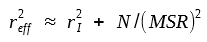where rI is the relative noise level in the absence of reflection feedback, N is the number of external-cavity modes, and MSR is the factor by which the external-cavity modes remain suppressed. The figure below shows the reflection-noise power penalty as a function of MSR for several values of N by choosing rI = 0.01.The power penalty is negligible in the absence of feedback (N = 0). However, it increases with an increase in N and a decrease in MSR. In fact, the penalty becomes infinite when MSR is reduced below a critical value. Thus, reflection feedback can degrade system performance to the extent that the system cannot achieve the desired BER despite an infinite increase in the power received. Such reflection-induced BER floors have been observed experimentally and indicate the severe impact of reflection noise on the performance of lightwave systems. An example of the reflection-induced BER floor is seen in the previous figure, where the BER remains above 10-9 for feedback levels in excess of -25 dB. Generally speaking, most lightwave systems operate satisfactorily when the reflection feedback is below -30 dB. In practice, the problem can be nearly eliminated by using an optical isolator within the transmitter module.

Even when an isolator is employed, reflection noise can be a problem for lightwave systems. In long-haul fiber links, fiber dispersion can convert phase noise into intensity noise, leading to performance degradation. Similarly, two reflecting surfaces anywhere along the fiber link act as a Fabry-Perot interferometer which can convert phase noise into intensity noise. Such a conversion can be understood by noting that multiple reflections inside a Fabry-Perot interferometer lead to a phase-dependent term in the transmitted intensity which fluctuates in response to phase fluctuations. As a result, the RIN of the signal incident on the receiver is higher than that occurring in the absence of  reflection feedback. Most of the RIN enhancement occurs over a narrow frequency band whose spectral width is governed by the laser linewidth (~100 MHz). Since total noise is obtained by integrating over the receiver bandwidth, it can affect system performance considerably at bit rates larger than 1 Gb/s. The power penalty can still by calculated usingA simple model that includes only two reflections between the reflecting interfaces shows that reff is proportional to (R1R2)1/2, where R1 and R2 are the reflectivities of the two interfaces.

Power penalty can become infinite and lead to BER floors when  reff exceeds 0.2. Such BER floors have been observed experimentally. They can be avoided only by eliminating or reducing parasitic reflections along the entire fiber link. It is therefore necessary to employ connectors and splices that reduce reflections through the use of index matching or other techniques.

Dispersion-induced pulse broadening affects the receiver performance in two ways. First, a part of the pulse energy spreads beyond the allocated bit slot and leads to intersymbol interference. Second, the pulse energy within the bit slot is reduced when the optical pulse broadens. Such a decrease in pulse energy reduces the SNR at the decision circuit. As the SNR should remain constant to maintain the system performance, the receiver requires more average power. This is the origin of dispersion-induced power penalty δd.

An exact calculation of δd is difficult, as it depends on many details such as the extent of pulse shaping at the receiver. A rough estimate can be obtained where broadening of Gaussian pulses is discussed. It shows that the optical pulse remains Gaussian, but is peak power is reduced by a pulse-broadening factor. If we define the power penalty δd as the increase (in decibels) in the received power that would compensate the peak-power reduction, δd is given by

δd = 10log10bf

where bf is the pulse broadening factor. We consider the case of broadband and narrowband optical sources separately.

Consider first a lightwave system designed with a relatively broadband optical source. The broadening factor bf has the form

bf = σ/σ0 = [1 + (DLσλ0)2 ]1/2

where σλ is the RMS width of the source spectrum. The RMS width σ0 of the optical pulse at the transmitter end is a design parameter. It can be related to the duty cycle dc of RZ pulses as 4σ0 = dcTb, where Tb ≡ 1/B is the duration of the bit slot at a given bit rate B. Using the two equations above, the power penalty becomes

δd = 5 log10 [1 + (4BLDσλ/dc)2]

If we assume that input pulses are wide enough to occupy the entire bit slot (dc = 1), the power penalty is negligible for 4BLDσλ << 1, becomes 1.5 dB when 4BLDσλ = 1, and increases rapidly beyond that.

In the case of a long-haul system designed with a narrowband source and chirp-free pulses, the broadening factor is obtained from the equation below: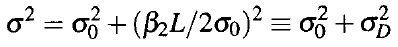If we use again σ0 = dc/(4B), the power penalty is given by

δd = 5 log10 [1 + (8β2B2L/dc2)2]

The figure below shows power penalty as a function of the dimensionless parameter combination μ = |β2|B2L for three values of dc.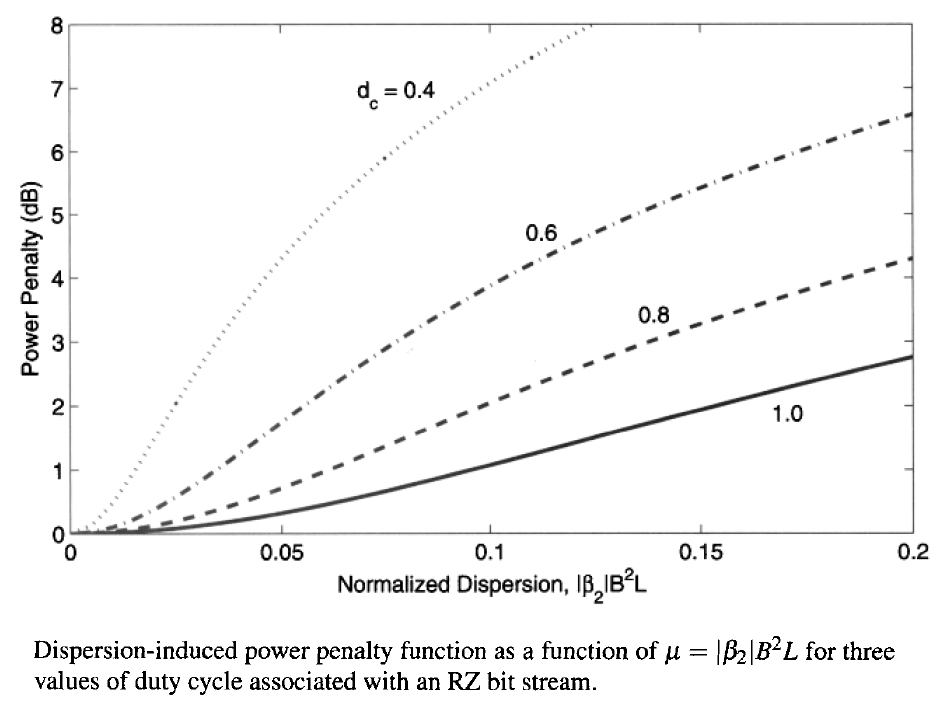Although the power penalty is negligible for values of μ < 0.05 and dc > 0.5, it increases rapidly as μ increases and exceeds 5 dB for μ = 0.1 and dc = 0.5. It is thus important to keep μ below 0.1. As an example, the operating distance of a 10-Gb/s lightwave system is limited to below 50 km because of dispersion when standard fibers are used with |β2| ≈ 20 ps2/km, but this value can be increased considerably through dispersion management. It should be stressed that the δd equation above provides only a rough estimate as its derivation is based on the assumptions of a Gaussian pulse shape.

#### 5. Frequency Chirping

The preceding discussion of dispersion-induced power penalty assumed that the input pulses were unchirped. An initial chirp on optical pulses is known to limit the performance of 1.55-μm lightwave systems when directly modulated semiconductor lasers are used to generate the digital bit stream. Frequency chirping can enhance the dispersion-induced broadening of optical pulses and thus may degrade the performance of a long-haul lightwave system more than that expected when unchirped pulses are employed.

An exact calculation of the chirp-induced power penalty δc is difficult because frequency chirp depends on both the shape and the width of the optical pulse. However, if we assume a Gaussian pulse shape and a linear chirp, we can do analysis to estimate the chirp-induced power penalty. If we use the following equation for pulse broadening factor:with

δc = 10log10bf

andthe power penalty is given by

δc = 5 log10 [(1 + 8Cβ2B2L/dc2)2 + (8β2B2L/dc2)2]

The following figure shows the chirp-induced power penalty as a function of |β2|B2L for several values of the chirp parameter C with dc = 1.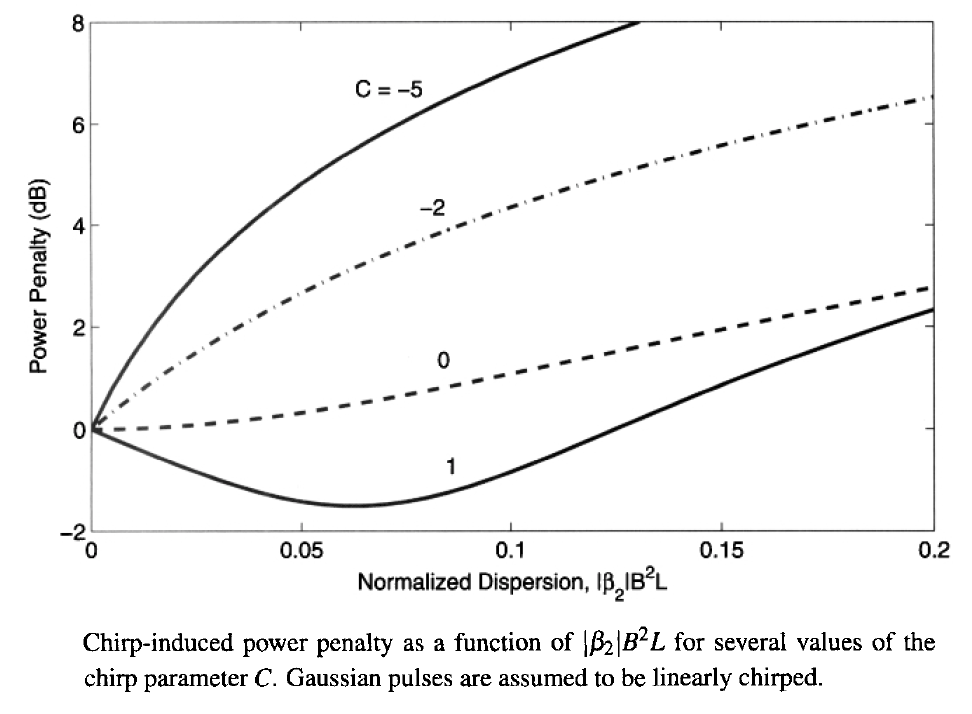The parameter β2 is taken to be negative, as is the case for 1.55-μm lightwave systems. The C = 0 curve corresponds to the case of a chirp-free pulse. Power penalty is negligible (<0.1 dB) in this ideal case as long as |β2|B2L < 0.05. However, the penalty can exceed 5 dB if the transmitted pulses are chirped such that C = -6 (a typical value for semiconductor lasers). To keep the penalty below 0.1 dB, the system should be designed with |β2|B2L < 0.002. For standard optical fibers with β2 ≈ -20 ps2/km, B2L is limited to 100 (Gb/s)2-km, indicating that even at B = 2.5 Gb/s, the transmission distance is limited to below 16 km because of frequency chirping. Interestingly, system performance can be improved by ensuring that β2C < 0. Each optical pulse then undergoes an initial compression phase. As C is negative for semiconductor lasers, fibers with "normal" dispersion (β2 > 0) can provide better performance whenever directly modulated semiconductor lasers are employed. For this reason, fibers with normal GVD are often employed in metropolitan area networks. Alternatively, one can make use of dispersion compensation and ensure that the average value of β2 is close to zero.

#### 6. Eye-Closure Penalty

An alternative measure of system performance is provided by the extent the "eye opening" in the eye diagram is affected by the dispersive and nonlinear effects accumulated inside the fiber link. An electrical filter with a bandwidth smaller than the bit rate is used inside the receiver to reshape pulses before they reach the decision circuit. The following figure shows the eye diagram looks like for an NRZ-format bit stream.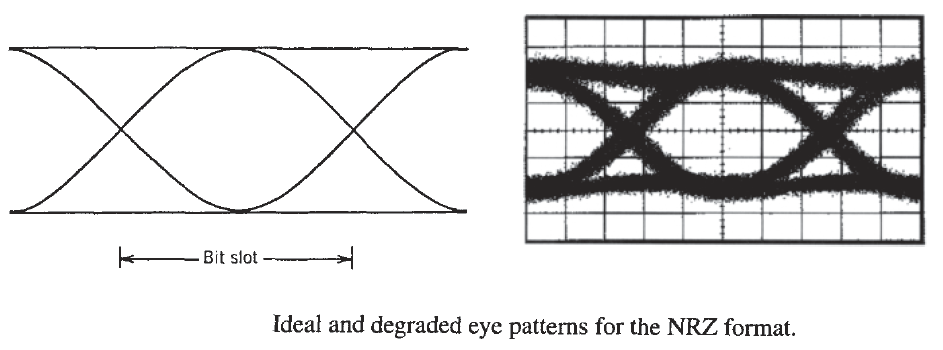When RZ format is employed, the pattern still has the appearance of an eye, even though the top horizontal rail is missing. Even in the case of the DPSK format, the eye diagram retains its shape. Look at the figure below.The top row shows the measured eye diagrams at 40 Gb/s in the case of NRZ, CSRZ, NRZ-DPSK, and RZ-DPSK formats under the back-to-back condition in which the transmitter is directly connected to the receiver.

As the optical bit stream is transmitted through a fiber link, the accumulation of dispersive and nonlinear effects distorts optical pulses. These distortions manifest in the eye diagram through a reduced opening of the eye. The bottom row shows the measured eye diagrams at 40 Gb/s after a 263-km-long fiber link for the same four modulation formats. As seen there, eye opening is reduced for all formats. Since the decision threshold is set in the center of the open portion of the eye, any reduction in eye opening indicates an increase in BER. This observation relates eye closure to the BER and suggests that its magnitude can provide a measure of the system performance. More precisely, the eye-closure penalty is quantified (in dB) as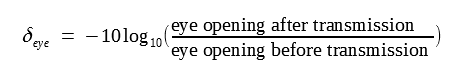Before this equation can be used, we need to clarify what one means by eye opening. Ideally, the eye amplitude peaks in the center of the bit slot and it provides an appropriate measure of eye opening. However, in practice, timing jitter makes it difficult to sample each pulse precisely at the location where pulse amplitude is maximum. If we allow an uncertainty of up to 10% on each side of the decision threshold, we should consider a rectangle of the largest area with a base of 0.2Tb, where Tb is the duration of each symbol, that can be fitted within the open portion of the eye. The height of this rectangle then measures eye opening. This approach is commonly adopted for numerical simulations.